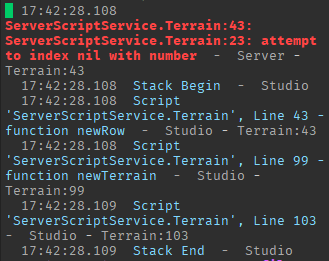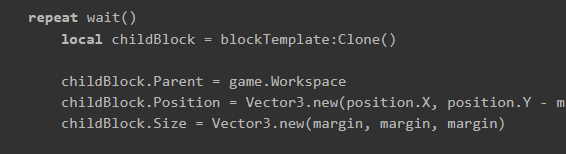# Help with coroutine

Hello,

I’m trying to make a blocky terrain like in Minecraft and I’m using coroutines to speed up the generation because I need to generate a lot of blocks. But when I run my script it gives me an error and I don’t know how to solve it.

Script:

``````-- @ Terrain

--// Variables

local replicatedStorage = game:GetService("ReplicatedStorage")
local assets = replicatedStorage:WaitForChild("Assets")
local blockTemplate = assets:WaitForChild("Block")
local treeTemplate = assets:WaitForChild("Tree")

--// Functions

function newRow(width, length, chunkHeight, margin, rowThreadCount, rowCount)
coroutine.wrap(function()
local treeOffset = math.random(50, 100)
local treeIndex = 0

for x = 1, width / rowThreadCount do
for z = 1, length do
local terrainBlock = blockTemplate:Clone()
local threadCount = chunkHeight / margin

terrainBlock.Parent = game.Workspace
terrainBlock.Position = Vector3.new((width * margin) / 2 - ((x + ((rowCount - 1) * (width / rowThreadCount))) * margin), math.floor(grid[x + ((rowCount - 1) * (width / rowThreadCount))][z] / margin) * margin + chunkHeight / 2, (length * margin) / 2 - z * margin)
terrainBlock.Size = Vector3.new(margin, margin, margin)

for count = 1, threadCount do
end

treeIndex += 1

if treeIndex == treeOffset then
local tree = treeTemplate:Clone()

tree.Parent = game.Workspace
tree:SetPrimaryPartCFrame(CFrame.new(Vector3.new((width * margin) / 2 - ((x + ((rowCount - 1) * (width / rowThreadCount))) * margin), math.floor(grid[x + ((rowCount - 1) * (width / rowThreadCount))][z] / margin) * margin + chunkHeight / 2 + blockTemplate.Size.Y, (length * margin) / 2 - z * margin)))

treeIndex = 0
treeOffset = math.random(50, 100)
end
end
end
end)()
end

function newColoumn(position, threadCount, margin, startPosition, maxHeight)
coroutine.wrap(function()
local blocks = {}
local blockIndex = startPosition
local lastChildBlock

repeat wait()
local childBlock = blockTemplate:Clone()

childBlock.Parent = game.Workspace
childBlock.Position = Vector3.new(position.X, position.Y - margin * blockIndex, position.Z)
childBlock.Size = Vector3.new(margin, margin, margin)

lastChildBlock = childBlock

table.insert(blocks, childBlock)
until lastChildBlock.Position.Y <= maxHeight

coroutine.wrap(function()
for _, block in pairs(blocks) do
if block.Position.Y < maxHeight then
block:Destroy()
end
end
end)()
end)()
end

function getPerlinNoise(width, length, margin, amplitude, seed)
local grid = {}

for x = 1, width do
grid[x] = {}

for z = 1, length do
grid[x][z] = math.noise(x / width, z / length, seed) * amplitude
end
end

return grid
end

function newTerrain(width, length, margin, amplitude, seed, chunkHeight)
local grid = getPerlinNoise(width, length, margin, amplitude, seed)

for rowCount = 1, rowThreadCount do
newRow(width, length, chunkHeight, margin, rowThreadCount, rowCount)
end
end

newTerrain(100, 100, 4, 150, 0, 256) -- | Make sure to keep the chunk height divisible by the margin for best performance
``````

Error:just a theory but you called the function with rowthreadcount as 0, and you divided with that value, making it so the division number doesnt exist

Can you tell us which line is 23?

Some changes I would recommend

For such performance-intensive code, you should probably use multiple scripts, placed under actors, for multi-threading.Use task.wait instead of wait, task.spawn instead of coroutine.wrap, and do the .Parent at the end of the properties. BulkMoveTo would also help.

No “rowThreadCount” is set to 2, “rowCount” is 0 but I only multiply with it.